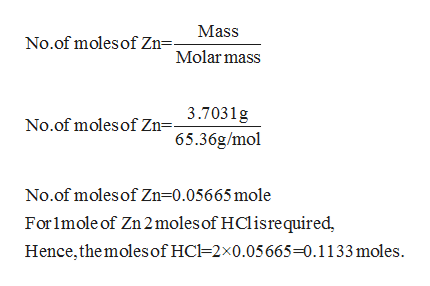# Zinc reacts with hydrochloric acid according to the reaction equation shown. Zn(s)+2HCl(aq)⟶ZnCl2(aq)+H2(g)Zn(s)+2HCl(aq)⟶ZnCl2(aq)+H2(g) How many milliliters of 4.504.50 M HCl(aq) are required to react with 7.557.55 g of an ore containing 49.049.0% Zn(s) by mass?volume: mL

Question

Zinc reacts with hydrochloric acid according to the reaction equation shown.

Zn(s)+2HCl(aq)⟶ZnCl2(aq)+H2(g)Zn(s)+2HCl(aq)⟶ZnCl2(aq)+H2(g)

How many milliliters of 4.504.50 M HCl(aq) are required to react with 7.557.55 g of an ore containing 49.049.0% Zn(s) by mass?

volume:

mL
check_circleExpert Solution
Step 1

The gram of Zn can be calculated as

Step 2

The reaction of zinc with hydrochloric acid is shown as,

Step 3

It is required to calculate the volume in mL of 4.50 M HCl which will react with 3.7031 g of Zinc. To c...help_outlineImage TranscriptioncloseMass No.of moles of Zn=- Molar mass 3.7031g No.of moles of Zn=- 65.36g/mol No.of moles of Zn 0.05665 mole For1mole of Zn 2 moles of HCl isrequired Hence,the moles of HC=2x0.05665-0.1133 moles. fullscreen

### Want to see the full answer?

See Solution

#### Want to see this answer and more?

Solutions are written by subject experts who are available 24/7. Questions are typically answered within 1 hour*

See Solution
*Response times may vary by subject and question
Tagged in

### Inorganic Chemistry